Win up to 100% scholarship on Aakash BYJU'S JEE/NEET courses with ABNAT Win up to 100% scholarship on Aakash BYJU'S JEE/NEET courses with ABNAT

# JEE Main 2022 July 25 – Shift 1 Chemistry Question Paper with Solutions

The JEE Main 2022 July 25 – Shift 1 Chemistry Question Paper with Solutions is given on this page. JEE Main 2022 question paper with solutions is given in a detailed manner so that students can easily understand the solutions. JEE Main 2022 answer keys are prepared by specialised experts at BYJU’S. Practising JEE Main 2022 July 25 – Shift 1 Chemistry Question Paper with Solutions will definitely enhance the confidence of the JEE aspirants.

## JEE Main 2022 25th July Shift 1 Chemistry Question Paper and Solutions

SECTION – A

Multiple Choice Questions: This section contains 20 multiple choice questions. Each question has 4 choices (1), (2), (3) and (4), out of which ONLY ONE is correct.

1. SO2Cl2 on reaction with excess of water results into acidic mixture

$$\begin{array}{l} SO_2Cl_2 + 2H_2O \rightarrow H_2SO_4 + 2HCl\end{array}$$

16 moles of NaOH is required for the complete neutralisation of the resultant acidic mixture. The number of moles of SO2Cl2 used is

(A) 16

(B) 8

(C) 4

(D) 2

Sol.

$$\begin{array}{l} SO_2Cl_2 + 2H_2O \rightarrow H_2SO_4 + 2HCl\end{array}$$

Moles of NaOH required for complete neutralisation of resultant acidic mixture = 16 moles

And 1 mole of SO2Cl2 produced 4 moles of H+.

$$\begin{array}{l}\therefore\text{Moles of}~SO_2Cl_2~ \text{used will be} = \frac{16}{4}= 4~ \text{moles}\end{array}$$

2. Which of the following sets of quantum numbers is not allowed?

$$\begin{array}{l} (\text{A})\ n=3, l=2, m_l=0, s=+\frac{1}{2} \end{array}$$
$$\begin{array}{l} (\text{B})\ n=3, l=2, m_l=-2, s=+\frac{1}{2} \end{array}$$
$$\begin{array}{l} (\text{C})\ n=3, l=3, m_l=-3, s=-\frac{1}{2} \end{array}$$
$$\begin{array}{l} (\text{D})\ n=3, l=0, m_l=0, s=-\frac{1}{2} \end{array}$$

Sol. If n = 3, then possible values of l = 0, 1, 2

But in option (C), the value of l is given ‘3’, this is not possible.

3. The depression in the freezing point observed for a formic acid solution of concentration 0.5 mL L–1 is 0.0405°C. Density of formic acid is 1.05 g mL–1. The Van’t Hoff factor of the formic acid solution is nearly (Given for water kf = 1.86 k kg mol–1)

(A) 0.8

(B) 1.1

(C) 1.9

(D) 2.4

Sol. ΔTf of formic acid = 0.0405°C

Concentration = 0.5 mL/L

and density = 1.05 g/mL

∴ Mass of formic acid in solution = 1.05 × 0.5 g

= 0.525 g

∴ According to Van’t Hoff equation,

$$\begin{array}{l}\triangle T_f = ik_f\cdot m\end{array}$$
$$\begin{array}{l} 0.0405=i\times 1.86\times \frac{0.525}{46\times 1}\end{array}$$

(Assuming mass of 1 L water = kg)

$$\begin{array}{l} i=\frac{0.0405\times 46}{1.86\times 0.525}=1.89\approx 1.9 \end{array}$$

4. 20 mL of 0.1 M NH4OH is mixed with 40 mL of 0.05 M HCl. The pH of the mixture is nearest to

(Given :Kb(NH4OH) = 1 × 10–5, log2 = 0.30, log3 = 0.48, log5 = 0.69, log7 = 0.84, log11 = 1.04)

(A) 3.2

(B) 4.2

(C) 5.2

(D) 6.2

Sol.

$$\begin{array}{l}\begin{matrix}NH_4OH & + & HCl & \longrightarrow & NH_4Cl+H_2O \\20\ mL, 0.1\ M & & 40\ mL, 0.05\ M & & \\2\ mmoles & & 2\ mmoles & & 2\ mmoles \\\end{matrix}\end{array}$$

∴ In final solution 2 millimoles of NH4Cl is present.

$$\begin{array}{l} \therefore \left[NH_4Cl\right]=\frac{1}{30}\text{ molar}\end{array}$$
$$\begin{array}{l} pH=\frac{1}{2}\left[pk_w-pk_b-logC\right]\end{array}$$
$$\begin{array}{l} =\frac{1}{2}\left[14-5-\left(-1.48\right)\right] = 5.24\end{array}$$

5. Match List-I with List-II.

List-I List-II
(A) N2(g) + 3H2(g) → 2NH3(g) (I) Cu
(B) CO(g) + 3H2(g) → CH4(g) + H2O(g) (II) Cu/ZnO – Cr2O3
(C) CO(g) + H2(g) → HCHO(g) (III) FexOy + K2O + Al2O3
(D) CO(g) + 2H2(g) → CH3OH(g) (IV) Ni

Choose the correct answer from the options given below :

(A) (A) – (II), (B) – (IV), (C) – (I), (D) – (III)

(B) (A) – (II), (B) – (I), (C) – (IV), (D) – (III)

(C) (A) – (III), (B) – (IV), (C) – (I), (D) – (II)

(D) (A) – (III), (B) – (I), (C) – (IV), (D) – (II)

Sol. Here, we have to match the reactions with their correct catalyst :

$$\begin{array}{l} (\text{A}) \ N_2\left(g\right)+3H_2\left(g\right)\xrightarrow[~~~~~]{Fe_xO_y+K_2O+Al_2O_3} 2NH_3\left(g\right)\end{array}$$
$$\begin{array}{l} (\text{B}) \ CO\left(g\right)+3H_2\left(g\right)\xrightarrow[~~~~~~]{Ni}CH_4\left(g\right)+H_2O\left(g\right)\end{array}$$
$$\begin{array}{l} (\text{C}) \ CO\left(g\right)+H_2\left(g\right)\xrightarrow[~~~~~~~~]{Cu}HCHO\left(g\right)\end{array}$$
$$\begin{array}{l} (\text{D}) \ CO\left(g\right)+2H_2\left(g\right)\xrightarrow[~~~~~~~~]{Cu/ZnO-Cr_2O_3}CH_3-OH\left(g\right)\end{array}$$

∴ Option (C) is correct option.

6. The IUPAC nomenclature of an element with electronic configuration [Rn] 5f146d17s2 is

(A) Unnilbium

(B) Unnilunium

(D) Unniltrium

Sol. The element with electronic configuration [Rn] 5f146d17s2 has atomic number → 103

∴ Its IUPAC name is :Unniltrium

7. The compound(s) that is(are) removed as slag during the extraction of copper is

(A) CaO

(B) FeO

(C) Al2O3

(D) ZnO

(E) NiO

Choose the correct answer from the options given below :

(A) (C), (D) only

(B) (A), (B), (E) only

(C) (A), (B) only

(D) (B) only

Sol. The compound(s) that are removed as a slag during the extraction of copper is :

$$\begin{array}{l} FeS\xrightarrow[~~~~~]{O_2/SiO_2}\underset{\text{slag}}{FeSiO_3}+SO_2\end{array}$$

∴ Only iron oxide (FeO) formed slag during extraction of copper.

8. The reaction of H2O2 with potassium permanganate in acidic medium leads to the formation of mainly

(A) Mn2+

(B) Mn4+

(C) Mn3+

(D) Mn6+

Sol. The reaction of KMnO4 with H2O2 in an acidic medium is as

$$\begin{array}{l}2KMnO_4 + 3H_2SO_4 + 5H_2O_2 \rightarrow K_2SO_4 + 2MnSO_4 + 8H_2O + 5O_2\end{array}$$

∴ Mn2+ will be formed as the product.

9. Choose the correct order of density of the alkali metals.

(A) Li < K < Na < Rb < Cs

(B) Li < Na < K < Rb < Cs

(C) Cs < Rb < K < Na < Li

(D) Li < Na < K < Cs < Rb

Sol. The increasing order of density of alkali metals as

$$\begin{array}{l} \underset{0.53}{Li}<\underset{0.86}{K}<\underset{0.97}{Na}<\underset{1.53}{Rb}<\underset{1.87}{CS}\underset{\text{(in g/dm}^3)}{}\end{array}$$

‘K’ metal has less density as compare to ‘Na’ metal.

10. The geometry around boron in the product ‘B’ formed from the following reaction is

$$\begin{array}{l} BF_3+NaH\xrightarrow[~~~~]{450\text{ K}}A+NaF\end{array}$$
$$\begin{array}{l} A+NMe_3\rightarrow B\end{array}$$

(A) Trigonal planar

(B) Tetrahedral

(C) Pyramidal

(D) Square planar

Sol.

$$\begin{array}{l} 2BF_3+6NaH\xrightarrow[~~~~~~~~]{450\text{ K}}\underset{(\text{A})}{B_2H_6}+6NaF\end{array}$$
$$\begin{array}{l} \underset{(\text{A})}{B_2H_6}+2NMe_3\rightarrow 2BH_3\cdot NMe_3\end{array}$$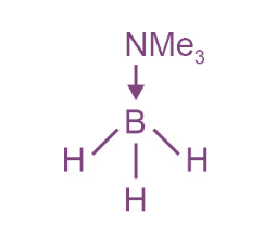∴ Geometry of boron will be tetrahedral.

11. The interhalogen compound formed from the reaction of bromine with excess of fluorine isa :

(A) hypohalite

(B) halate

(C) perhalate

(D) halite

Sol.

$$\begin{array}{l} Br_2+\underset{(\text{Excess})}{5F_2}\rightarrow 2BrF_5\end{array}$$

If BrF5 undergoes hydrolysis it will produce halide.

12. The photochemical smog does not generally contain :

(A) NO

(B) NO2

(C) SO2

(D) HCHO

Sol. Photochemical smog contain:

Ozone, nitric oxide, organic compounds, nitrogen dioxide, formaldehyde.

∴ SO2 is not the part of photochemical smog.

13. A compound’A’ on reaction with ‘X’ and ‘Y’ produces the same major product but differentby product’a’ and ‘b’. Oxidation of’a’ gives a substance produced by ants.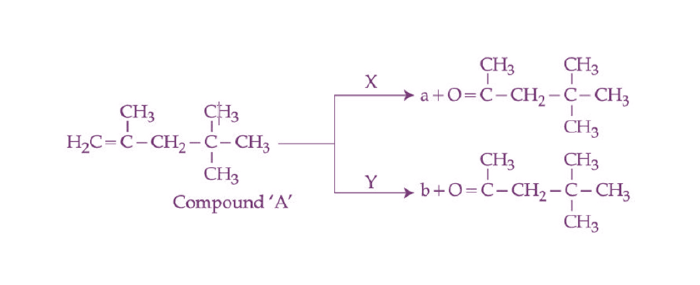‘X’ and ‘Y’ respectively are

(A) KMnO4/H+ and dil. KMnO4, 273 K

(B) KMnO4(dilute), 273 Kand KMnO4/H+

(C) KMnO4/H+ and O3, H2O/Zn

(D) O3, H2O/Zn and KMnO4/H+

Sol.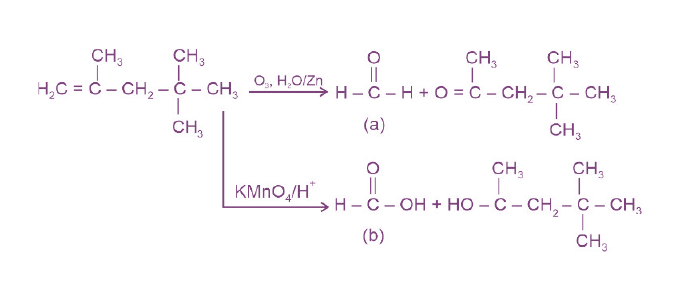* Ants produces formic acid in their venom gland.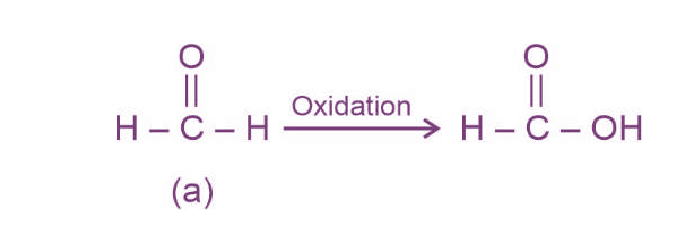14. Most stable product of the following reaction is :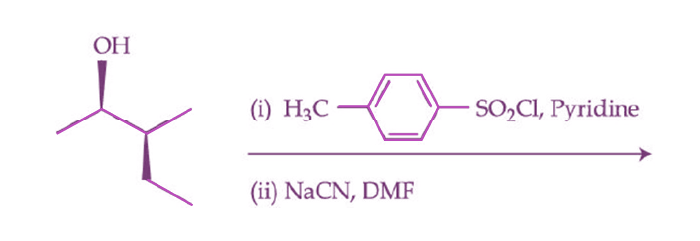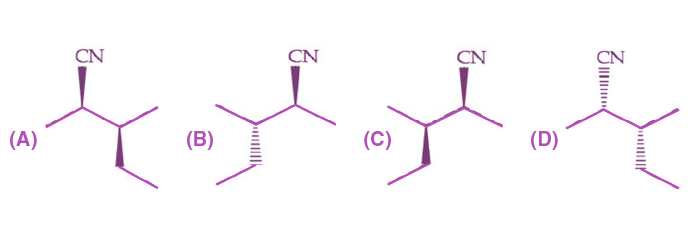Sol.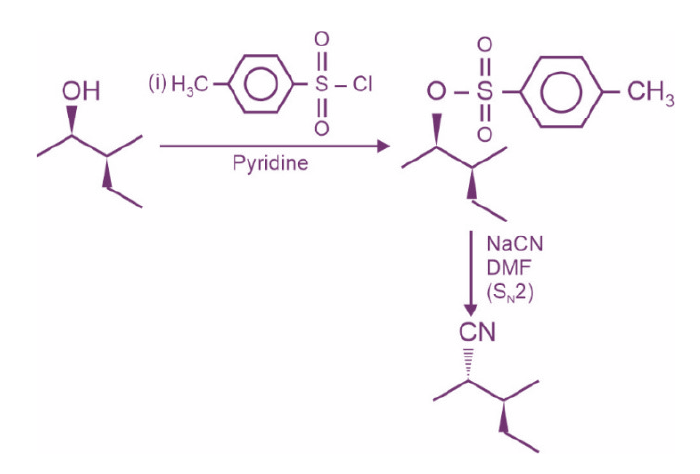∴ Option (B) is correct option.

15. Which one of the following reactions does not represent correct combination of substrateand product under the given conditions?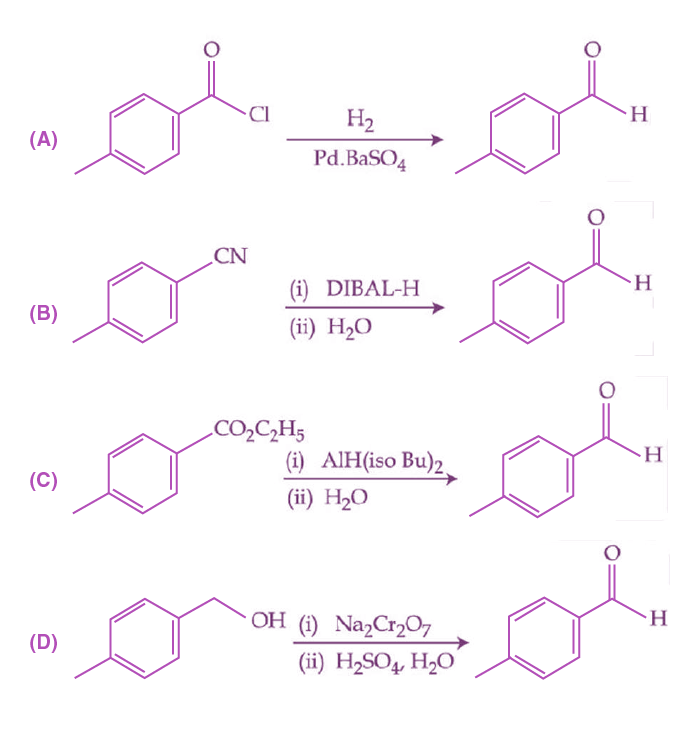Sol.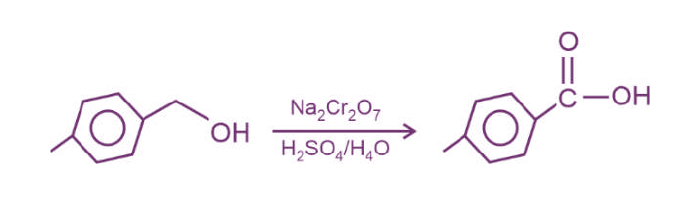Na2Cr2O7, H2SO4/H2O is the strongest oxidising agent and it will oxidise 1° alcohol into acids.

16. An organic compound ‘A’ on reaction with NH3 followed by heating gives compound B. Which on further strong heating gives compound C(C8H5NO2). Compound C on sequential reaction with ethanolic KOH, alkyl chloride and hydrolysis with alkali gives a primary amine. The compound A is :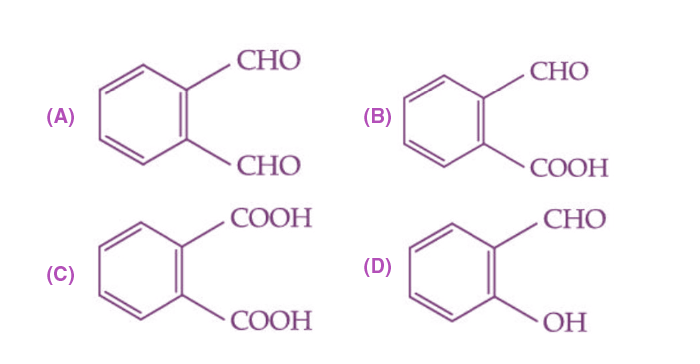Sol.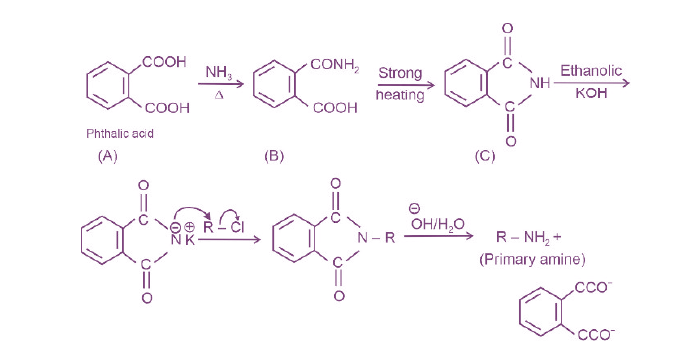All the given reactions can be explained if organic compound (A) is phthalic acid.

17. Melamine polymer is formed by the condensation of :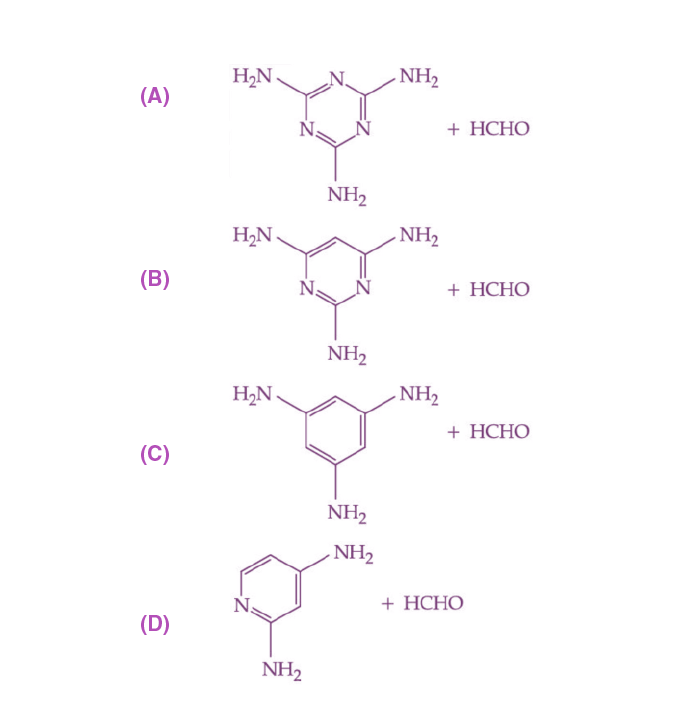Sol. Melamine polymer is formed by the condensation polymerisation of melamine and formaldehyde.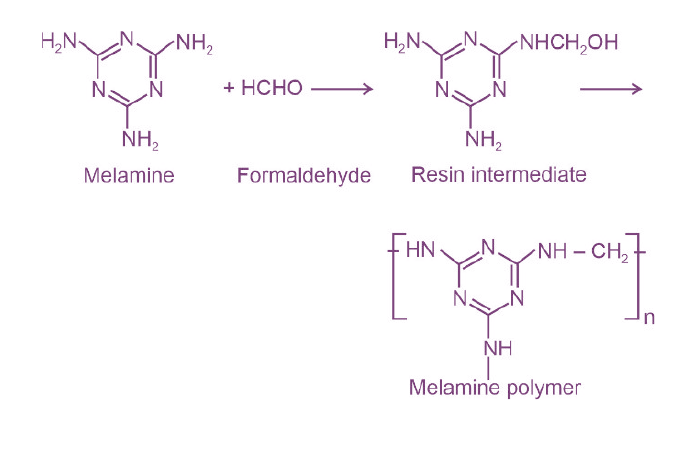18. During the denaturation of proteins, which of these structures will remain intact?

(A) Primary

(B) Secondary

(C) Tertiary

(D) Quaternary

Sol. During the denaturation of proteins hydrogen bonds are disturbed. As a result, the secondary and tertiary structures are destroyed but the primary structures remain intact.

19. Drugs used to bind to receptors, inhibiting its natural function and blocking a message are called:

(A) Agonists

(B) Antagonists

(C) Allosterists

(D) Anti histaminists

Sol. Drugs that bind to the receptor site and inhibit its natural function are called Antagonists.

20. Given below are two statements:

Statement I: On heating with KHSO4, glycerol is dehydrated and acrolein is formed.

Statement II: Acrolein has fruity odour and can be used to test glycerol’s presence.

Choose the correct option.

(A) Both Statement I and Statement II are correct.

(B) Both Statement I and Statement II are incorrect.

(C) Statement I is correct but Statement II is incorrect.

(D) Statement I is incorrect but Statement II is correct.

Sol. Glycerol, on heating with KHSO4, undergoes dehydration to give unsaturated aldehyde called acrolein. So, statement I is correct.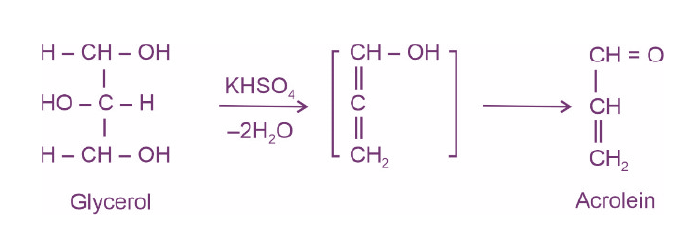Acrolein has piercing unpleasant smell. So, statement-II is incorrect.

SECTION – B

Numerical Value Type Questions: This section contains 10 questions. In Section B, attempt any five questions out of 10. The answer to each question is a NUMERICAL VALUE. For each question, enter the correct numerical value (in decimal notation, truncated/rounded-off to the second decimal place; e.g. 06.25, 07.00, –00.33, –00.30, 30.27, –27.30) using the mouse and the on-screen virtual numeric keypad in the place designated to enter the answer.

1. Among the following species

$$\begin{array}{l} N_2,N_2^+,N_2^-,N_2^{2-},O_2,O_2^+,O_2^-,O_2^{2-} \end{array}$$

the number of species showing diamagnetism is ________.

Sol. According to molecules orbital theory. The electronic configurations of the given species are

$$\begin{array}{l} N_2:\sigma1s^2\sigma^\ast1s^2\sigma2s^2\sigma^\ast2s^2\pi2p_x^2=\pi2p_y^2\sigma 2p_z^2 \end{array}$$
$$\begin{array}{l} N_2^+:\sigma1s^2\sigma^\ast1s^2\sigma2s^2\sigma^\ast2s^2\pi2p_x^2=\pi2p_y^2\sigma 2p_z^1 \end{array}$$
$$\begin{array}{l} N_2^-:\sigma1s^2\sigma^\ast1s^2\sigma2s^2\sigma^\ast2s^2\sigma2p_z^2\pi2p_x^2=\pi2p_y^2\pi^\ast 2p_x^1 \end{array}$$
$$\begin{array}{l} N_2^{2-}:\sigma1s^2\sigma^\ast1s^2\sigma2s^2\sigma^\ast2s^2\sigma2p_z^2\pi2p_x^2=\pi2p_y^2\pi^\ast 2p_x^1 =\pi^\ast2p_y^1\end{array}$$
$$\begin{array}{l} O_2:\sigma1s^2\sigma^\ast1s^2\sigma2s^2\sigma^\ast2s^2\sigma2p_z^2\pi2p_x^2=\pi2p_y^2\pi^\ast 2p_x^1 =\pi^\ast2p_y^1\end{array}$$
$$\begin{array}{l} O_2^+:\sigma1s^2\sigma^\ast1s^2\sigma2s^2\sigma^\ast2s^2\sigma2p_z^2\pi2p_x^2=\pi2p_y^2\pi^\ast 2p_1^x \end{array}$$
$$\begin{array}{l} O_2^-:\sigma1s^2\sigma^\ast1s^2\sigma2s^2\sigma^\ast2s^2\sigma2p_z^2\pi2p_x^2=\pi2p_y^2\pi^\ast 2p_x^2=\pi^\ast2p_y^1\end{array}$$
$$\begin{array}{l} O_2^{2-}:\sigma1s^2\sigma^\ast1s^2\sigma2s^2\sigma^\ast2s^2\sigma2p_z^2\pi2p_x^2=\pi2p_y^2\pi^\ast 2p_x^2=\pi^\ast2p_y^2\end{array}$$

Diamagnetic species are N2 and O22-

∴ Number of species showing diamagnetism = 2

2. The enthalpy of combustion of propane, graphite and dihydrogen at 298 K are –2220.0 kJ mol–1, –393.5 kJ mol–1 and –285.8 kJ mol–1, respectively. The magnitude of enthalpy of formation of propane (C3H8) is _____ kJ mol–1. (Nearest integer)

Sol. Enthalpy of combustion of propane, graphite and H2 at 298K are

$$\begin{array}{l} C_3H_8\left(g\right)+5O_2\left(g\right)\rightarrow 3CO_2\left(g\right)+4H_2O\left(I\right),\Delta H_1=-2220\text{ kJ mol}^{-1}\end{array}$$
$$\begin{array}{l} C\left(\text{graphite}\right)+O_2\left(g\right)\rightarrow CO_2\left(g\right),\Delta H_2=-393.5\text{ kJ mol}^{-1}\end{array}$$
$$\begin{array}{l} H_2\left(g\right)+\frac{1}{2}O_2\left(g\right)\rightarrow H_2O\left(l\right),\Delta H_3=-285.8\text{ kJ mol}^{-1}\end{array}$$

The desired reaction is

$$\begin{array}{l}3C(graphite) + 4H_2(g) \rightarrow C_3H_8(g)\end{array}$$
$$\begin{array}{l}\Delta H_f = 3\Delta H_2 + 4\Delta H_3 – \Delta H_1\end{array}$$
$$\begin{array}{l}= 3(-393.5) + 4(-285.8) – (-2220)\end{array}$$
$$\begin{array}{l}= -103.7~ \text{kJ mol}^{-1}\end{array}$$
$$\begin{array}{l} \left|\Delta H_f\right|\simeq 104\text{ kJ mol}^{-1}\end{array}$$

3. The pressure of a moist gas at 27°C is 4 atm. The volume of the container is doubled at the same temperature. The new pressure of the moist gas is ______ ×10–1 atm. (Nearest integer)

(Given: The vapour pressure of water at 27°C is 0.4 atm.)

Sol. From ideal gas equation,

$$\begin{array}{l} P\propto\frac{1}{V} \end{array}$$

P1V1 = P2V2

Pressure of the gas = 4 – 0.4 = 3.6 atm

3.6 V1 = P2 (2V1)

P2 = 1.8 atm

Hence, new pressure of moist gas is
1.8 + 0.4 = 2.2 atm = 22 × 10–1atm

4. The cell potential for Zn|Zn2+(aq)||Snx+|Sn is 0.801 V at 298 K. The reaction quotient for the above reaction is 10–2. The number of electrons involved in the given electrochemical cell reaction is _____.

$$\begin{array}{l} \left(\text{Given:}E^\circ_{Zn^{2+}|Zn}=-0.763V,E^\circ_{Sn^{x+}|Sn}=+0.008~\text{V}\text{ and }\frac{2.30 3RT}{F}=0.06 ~\text{V}\right)\end{array}$$

Sol.

$$\begin{array}{l}A: Zn \rightarrow Zn^{2+} + 2e^- \\C: Sn^{x+} + xe^-\rightarrow Sn\end{array}$$
$$\begin{array}{l} E_{\text{Cell}}^\circ=E_{Zn|Zn^{2+}}^\circ+E_{Sn^{x+}|Sn}^\circ\end{array}$$

⇒ 0.763 + 0.008 = 0.771 V

From Nernst equation,

$$\begin{array}{l} E_{\text{Cell}}=E_{\text{Cell}}^\circ\frac{-2.303 RT}{nF}\text{logQ} \end{array}$$
$$\begin{array}{l} 0.801=0.771-\frac{0.06}{n}\text{log}10^{-2} \end{array}$$
$$\begin{array}{l} 0.03=\frac{0.06}{n}\times 2\\n = 4\end{array}$$

5. The half-life for the decomposition of gaseous compound A is 240 s when the gaseous pressure was 500 torr initially. When the pressure was 250 torr, the half-life was found to be 4.0 min. The order of the reaction is ______. (Nearest integer)

Sol.

$$\begin{array}{l} \left(t_{1/2}\right)_A=240~\text{ s}~\text{when P} = 500~\text{torr} \end{array}$$

$$\begin{array}{l} \left(t_{1/2}\right)_A = 4~\text{minutes} = 4 \times 60 = 240~\text{seconds}~\text{when P }= 250~\text{torr}\end{array}$$

If means half-life is independent of concentration of reactant present.

∴ Order of reaction = 1

6. Consider the following metal complexes:

[Co(NH3)6]3+

[CoCl(NH3)5]2+

[Co(CN)6]3–

[Co(NH3)5(H2O)]3+

The spin-only magnetic moment value of the complex that absorbs light with shortest wavelength is _______ B.M. (Nearest integer)

Sol. In all complexes, Co is present in +3 oxidation state and all complexes are low spin or inner orbital complex.

Stronger the ligand, higher the crystal field splitting.

So, order of crystal field splitting is

[Co(CN)6]3– > [Co(NH3)6]3+> [Co(NH3)5(H2O)]3+ > [CoCl(NH3)5]2+.

Shortest wavelength is shown by complex having maximum crystal field splitting.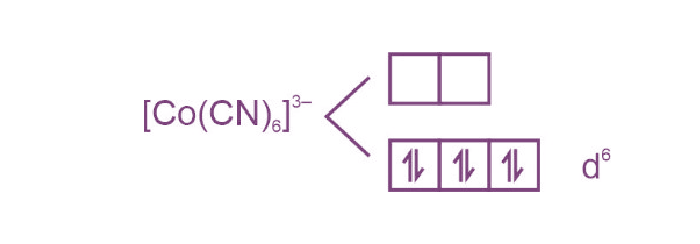$$\begin{array}{l}\text{Spin only magnetic moment} = \sqrt{0\left(0+2\right)}=0\text{ B.M} \end{array}$$

7. Among Co3+, Ti2+, V2+ and Cr2+ ions, one if used as a reagent cannot liberate H2 from dilute mineral acid solution, its spin-only magnetic moment in gaseous state is________ B.M. (Nearest integer)

Sol. Co3+ will not liberate H2 gas an reaction with dilute acid

$$\begin{array}{l} E_{Co^{3+}/Co^{2+}}^\circ =+1.97\end{array}$$

And Co3+ has electronic configuration = [Ar] 3d6

$$\begin{array}{l}\therefore \text{four unpaired electrons are present in it}\\ \therefore \text{Spin-only magnetic moment} = \sqrt{4\left(4+2\right)}\\=4.92\approx5 \end{array}$$

8. While estimating the nitrogen present in an organic compound by Kjeldahl’s method, the ammonia evolved from 0.25 g of the compound neutralized 2.5 mL of 2 M H2SO4. The percentage of nitrogen present in organic compound is ________.

Sol. NH3 gas is neutralized by 2⋅5 mL of 2 M H2SO4

∴ Moles of NH3 neutralized = 2⋅5 × 2 × 2 millimole

= 10×10–3 moles

∴ Weight of N present in compound will be

= 10 × 10–3× 14

= 0⋅14 g

• % of ‘N’ in compound
$$\begin{array}{l}=\frac{0.14}{0.25}\times 100= 56\%\end{array}$$

9. The number of sp3 hybridised carbons in an acyclic neutral compound with molecular formula C4H5N is ________.

Sol. C4H5N

$$\begin{array}{l}DBE= \left(C+1\right)-\left(\frac{H+X-N}{2}\right)\end{array}$$
$$\begin{array}{l} =\left(4+1\right)-\left(\frac{5-1}{2}\right)=5-2=3\end{array}$$

Three double bond equivalent are present in compound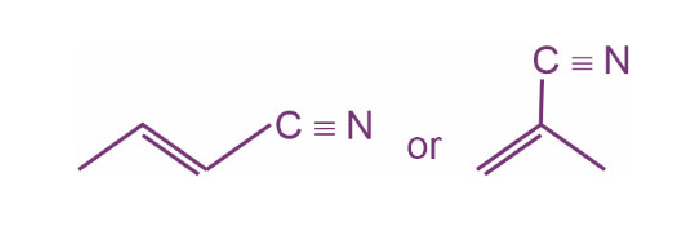Only1 sp3 hybridised carbon is there

(Keeping compound as acyclic)

10. In the given reaction,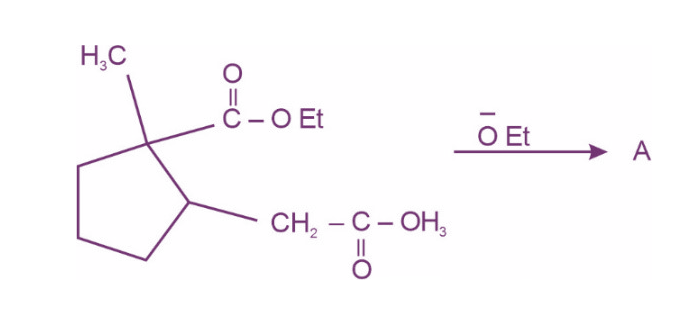(where Et is –C2H5)

The number of chiral carbon(s) in product A is ______.

Sol.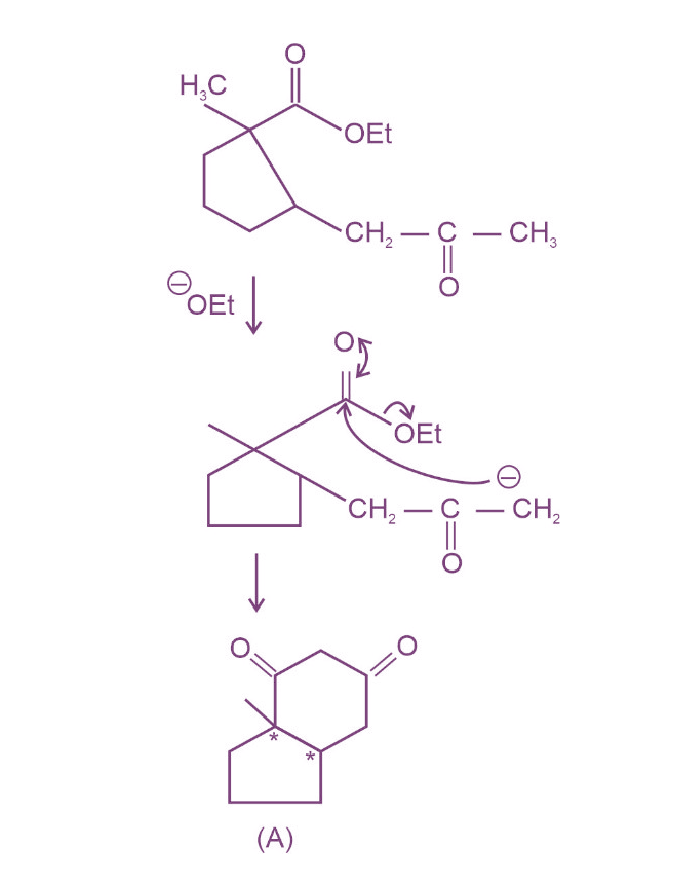Two chiral carbons are there in product A.

### Download PDF of JEE Main 2022 July 25 Shift 1 Chemistry Paper & Solutions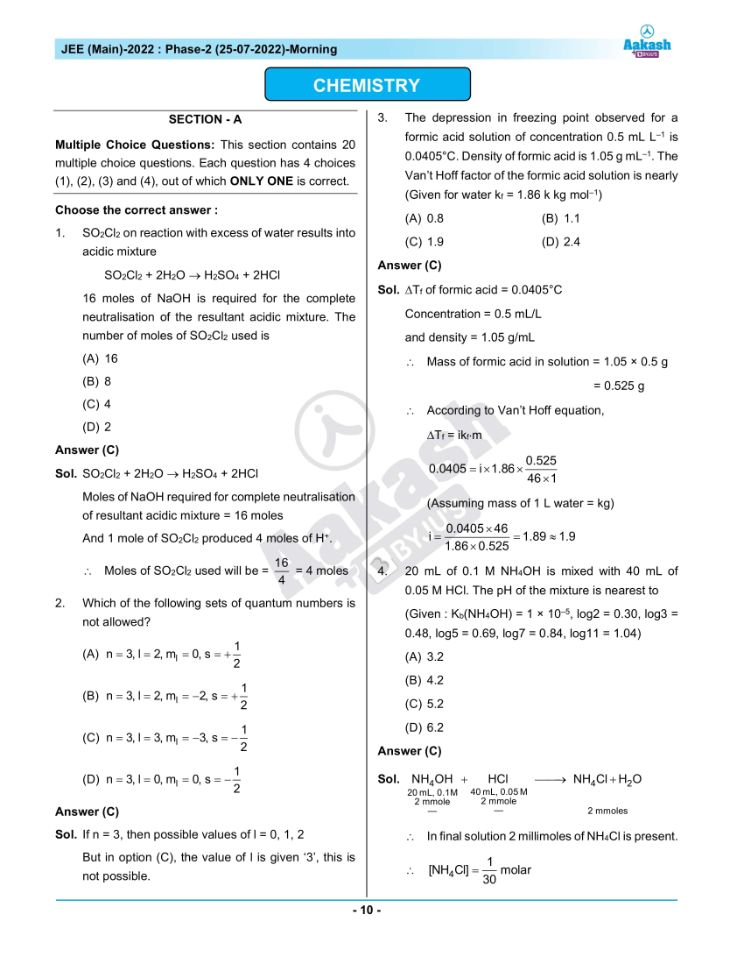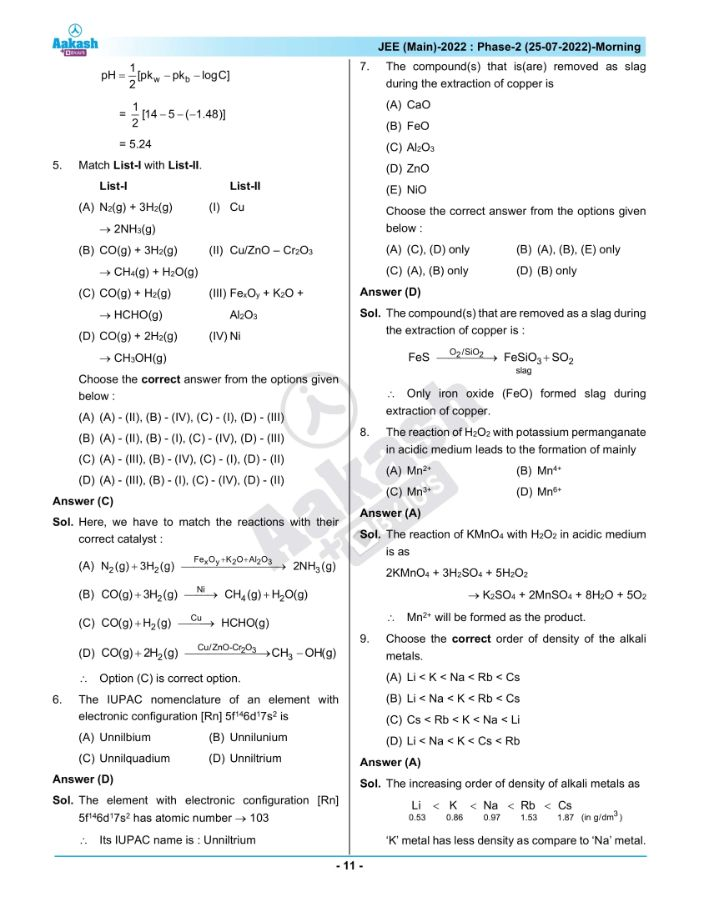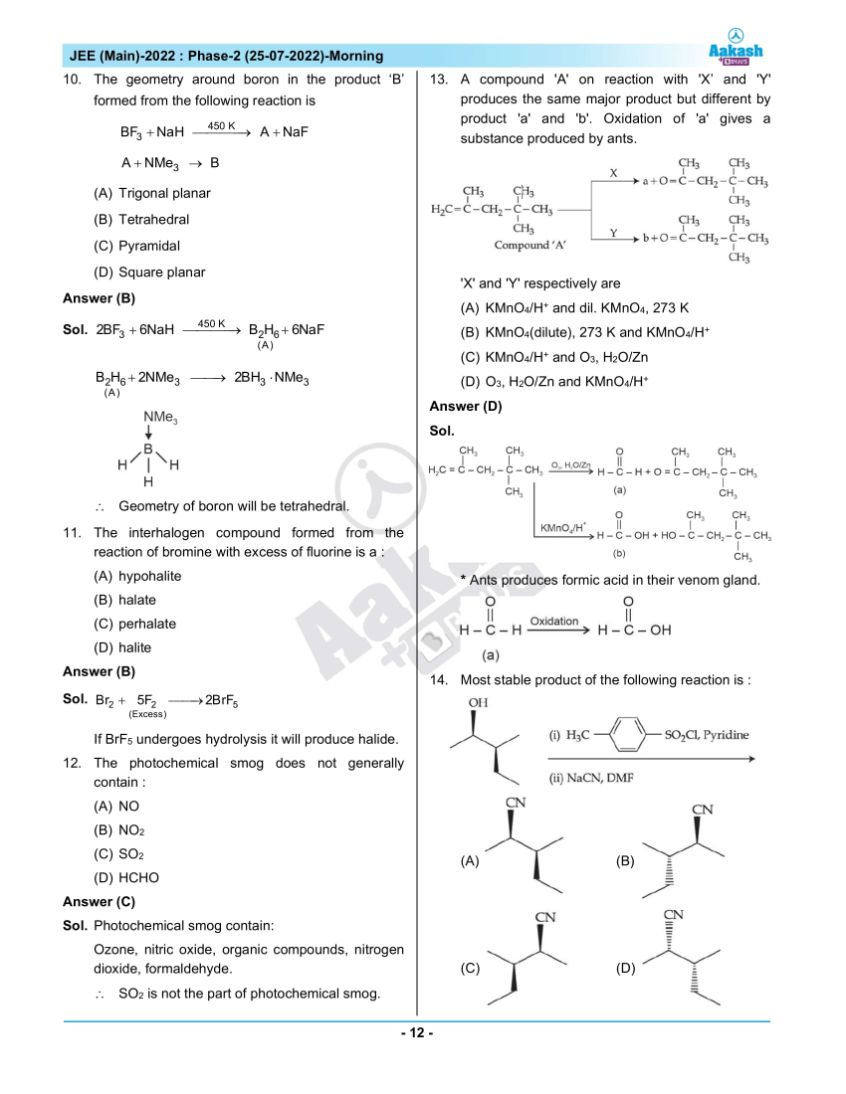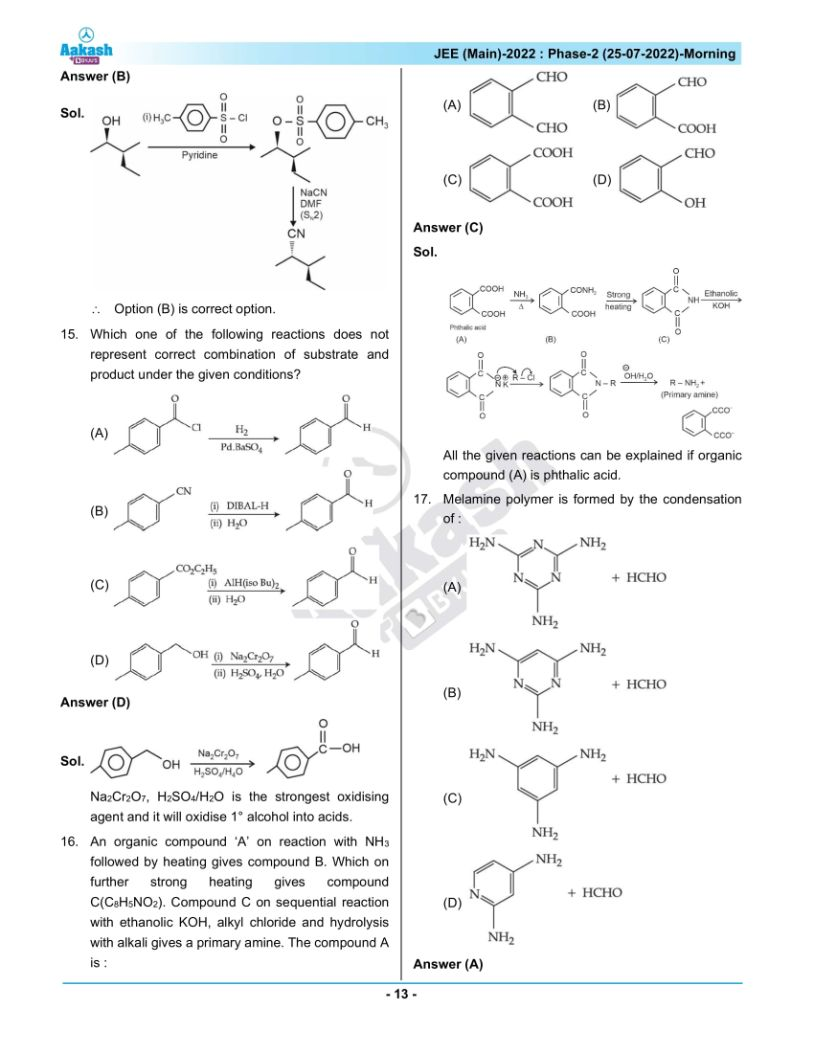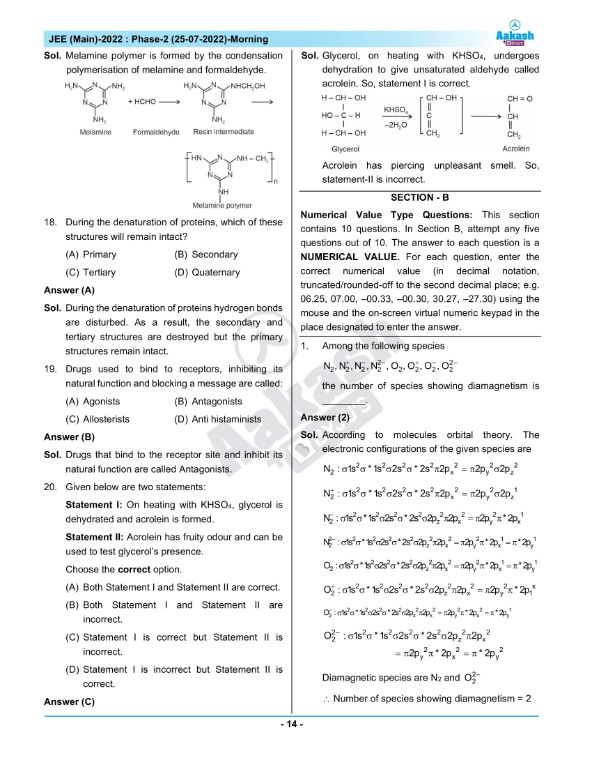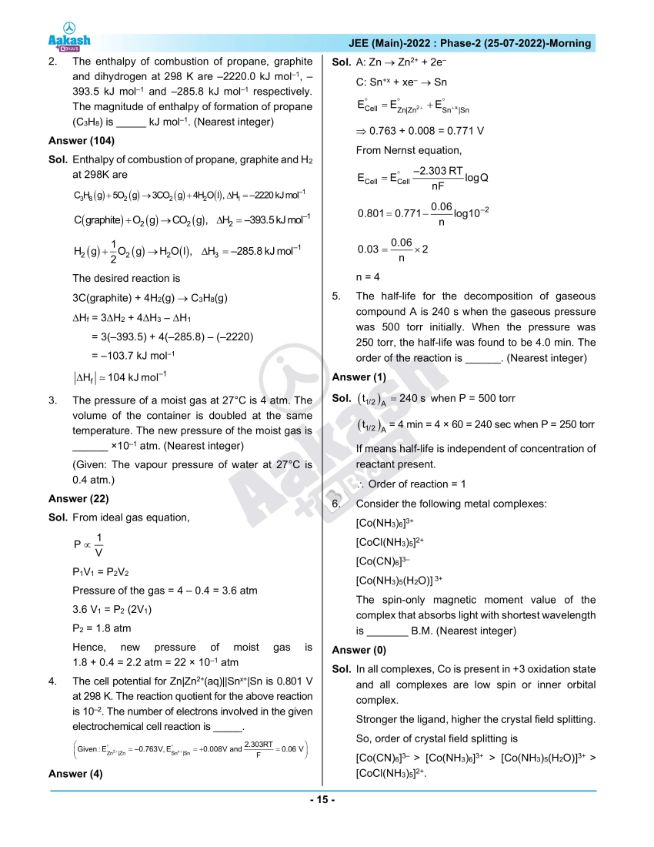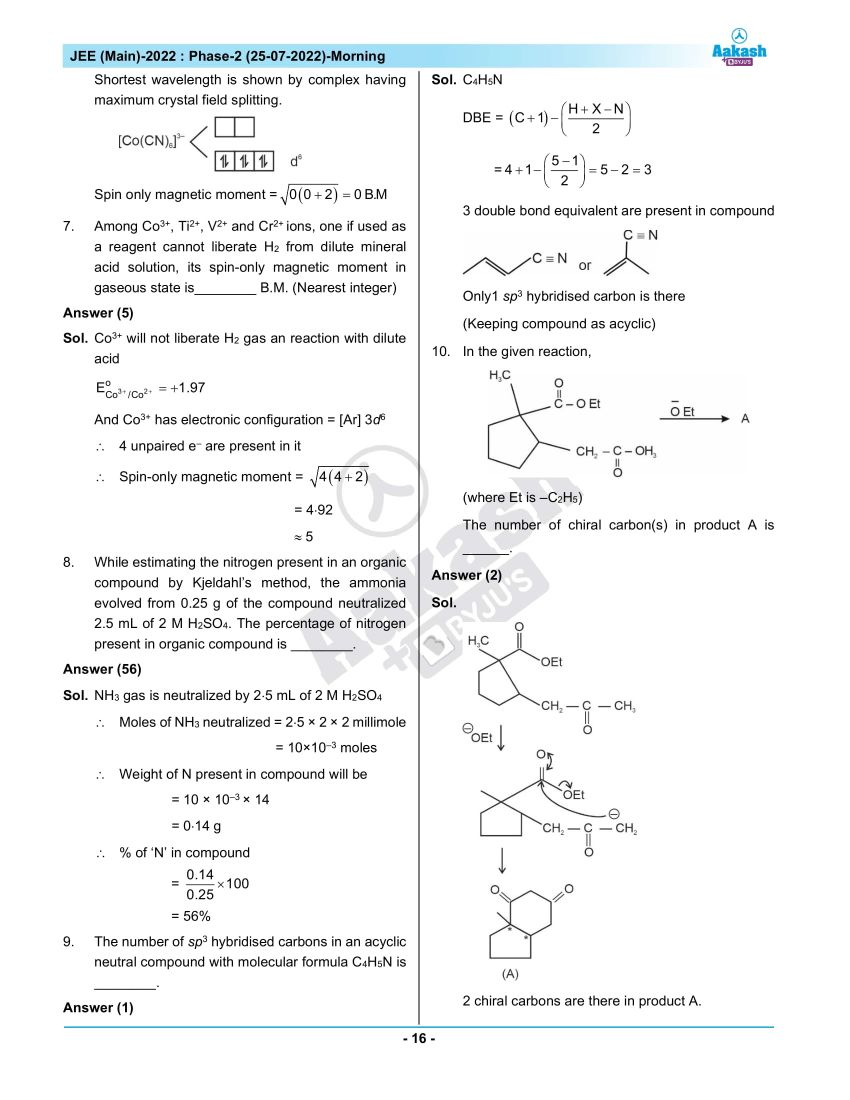## JEE Main 2022 July 25th Shift 1 Paper Analysis